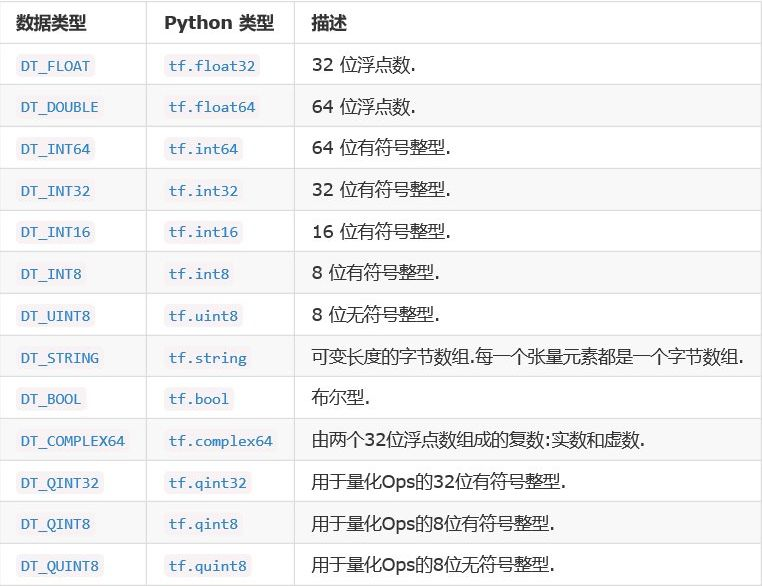# 机器学习篇(七)1、随即在数据中抽取x个样本，当做x个类别的中心点

2、计算其他点分别到这三个点的距离(欧氏距离),距离那个中心点近就划分为那个类别

3、计算每个类别的平均值，这个这个值于中心点相同，结束聚类。

sklearn.cluster.KMeans(）

import tensorflow as tf
hello = tf.constant('Hello, TensorFlow!')
sess = tf.Session()
print(sess.run(hello))
# 输出：Hello, TensorFlow!代表安装成功。


operstion(op):运算的操作节点,（所有的操作都是一个op）,例如上面的hello就是一个op。

Tensorflow是计算密集型的框架而Django,Flask,Scrapy是IO密集型框架。

1、tf.get_default_graph()

2、op、sess、tensor的graph属性。

import tensorflow as tf
a = tf.constant("Hello, TensorFlow!")
b = tf.constant("Hello, Python")
# 查看默认的图
graph = tf.get_default_graph()
print("graph:",graph)
with tf.Session() as sess:
print(a.graph)
print(b.graph)
print(sess.graph)
'''

<tensorflow.python.framework.ops.Graph object at 0x00000273EFC99908>
<tensorflow.python.framework.ops.Graph object at 0x00000273EFC99908>
<tensorflow.python.framework.ops.Graph object at 0x00000273EFC99908>
'''


# 创建一个图

import tensorflow as tf
g = tf.Graph()
print(g)
# 使用该图
with g.as_default():
s = tf.constant(1.0)
print(s.graph)
'''

<tensorflow.python.framework.ops.Graph object at 0x000001A758ED5518>
<tensorflow.python.framework.ops.Graph object at 0x000001A758ED5518>
'''


op：

op和tensor组成一张图。

op：只要使用了tensorflow里定义的函数定义的都是op

tensor(张量):代指数据

op中装tensor。

1、运行图的结构

2、分配资源计算

3、掌握资源

tf.Session()运行的是默认的图。

tf.Session(graph=g)运行g图。

sess = tf.Session()

sess.run()相当于启动整个图。

sess.close()在计算结束需要释放内存。用with就可以不用写了。

fetches:运行op或者计算tensor,可以列表，元组

feed_dict：用于实时提供数据，相当于一个占位符，运行的时候可以指定参数。graph:张量所在的图

op：张量的操作名

name：张量的字符串描述

shape：张量的形状

# 0维:()  1维:(x)  2维:(x,y) 3维:(x,y,z)

tf.Tensor.get_shape:获取静态形状

tf.Tensor.set_shape:改变Tensor的形状

# 创建一个张量,可以多行4列的数据

plt = tf.placeholder(tf.float32,[None,4])

print(plt)

# 改变形状

plt.set_shape([3,4])

print(plt)

# 再次改变会报错

plt.set_shape([2,6])

print(plt)

'''

Tensor("Placeholder:0", shape=(?, 4), dtype=float32)

Tensor("Placeholder:0", shape=(3, 4), dtype=float32)

'''

tf.reshape()：创建一个新张量。

tf_reshape = tf.reshape(plt,[2,6])

print(tf_reshape )

'''

Tensor("Reshape:0", shape=(2, 6), dtype=float32)

'''09-101560
01-2083009-2844万+
12-1416万+
09-15997
07-215万+
01-161万+
11-011万+
09-152795
11-032万+
07-199241
05-286299
08-194万+
04-05337
01-011181
06-151733
07-284万+
07-043666
11-171901
04-152676
©️2020 CSDN 皮肤主题: 深蓝海洋 设计师:CSDN官方博客